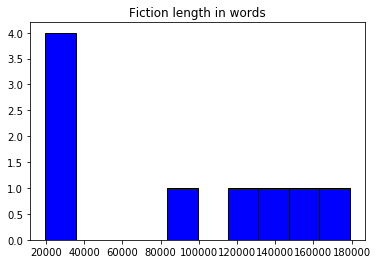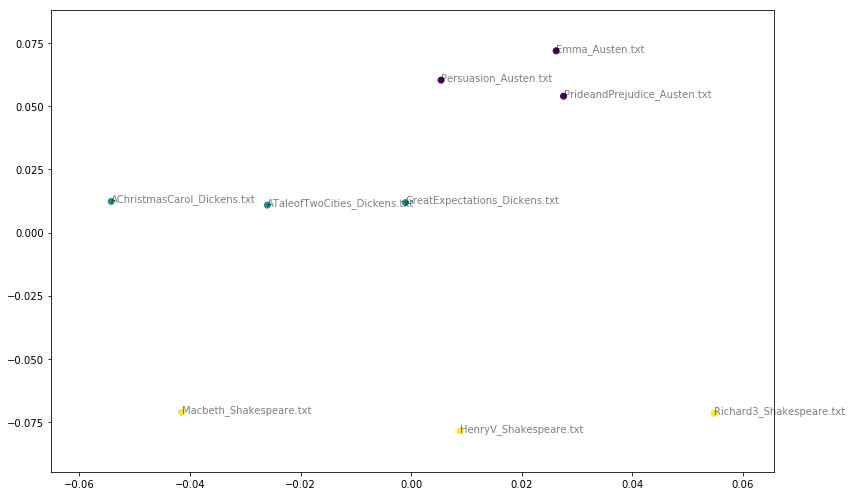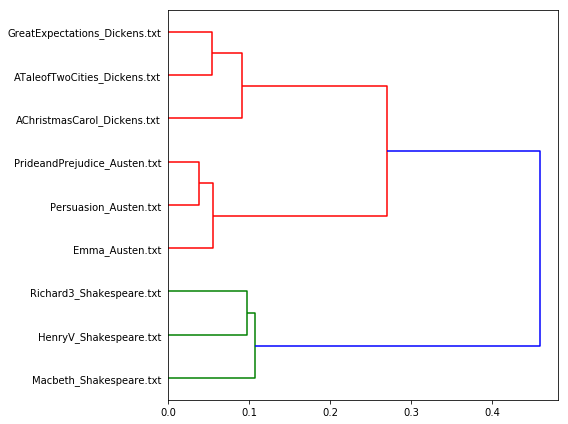The documents to be explored are from fictions of three authors.

• AChristmasCarol_Dickens.txt
• ATaleofTwoCities_Dickens.txt
• GreatExpectations_Dickens.txt
• Emma_Austen.txt
• Persuasion_Austen.txt
• PrideandPrejudice_Austen.txt
• HenryV_Shakespeare.txt
• Macbeth_Shakespeare.txt
• Richard3_Shakespeare.txt
• We will first take a look at the length of each text.
• Next, we will measure and visualize the distance of the texts.
• Last, we will generate a dendrogram to show the authorship grouping.
In :
import os
import numpy as np
import sklearn.feature_extraction.text as text

data_dir = 'Fictions/'

filenames = np.array(sorted(os.listdir(data_dir)))

filenames_with_path = [os.path.join(data_dir, fn) for fn in filenames] # join the path with filenames
In :
filenames_with_path
Out:
['Fictions/AChristmasCarol_Dickens.txt',
'Fictions/ATaleofTwoCities_Dickens.txt',
'Fictions/Emma_Austen.txt',
'Fictions/GreatExpectations_Dickens.txt',
'Fictions/HenryV_Shakespeare.txt',
'Fictions/Macbeth_Shakespeare.txt',
'Fictions/Persuasion_Austen.txt',
'Fictions/PrideandPrejudice_Austen.txt',
'Fictions/Richard3_Shakespeare.txt']
In :
vectorizer = text.CountVectorizer(input='filename')
In :
dtm = vectorizer.fit_transform(filenames_with_path)
In :
vocab = np.array(vectorizer.get_feature_names())
In :
# sum over rows to calculate lengths(the numbers in a row are word frequency of a document,so sum the numbers to get the word count of each document)
lengths = np.sum(dtm, axis=1)
print lengths
[[ 31549]



[ 28605]
[ 19700]
[ 83924]

[ 33849]]
In :
# plot histogragh
import matplotlib.pyplot as plt

plt.hist(lengths,edgecolor='black',facecolor='blue')
Out:
(array([ 4.,  0.,  0.,  0.,  1.,  0.,  1.,  1.,  1.,  1.]),
array([  19700. ,   35638.3,   51576.6,   67514.9,   83453.2,   99391.5,
115329.8,  131268.1,  147206.4,  163144.7,  179083. ]),
<a list of 10 Patch objects>)
In :
plt.title("Fiction length in words")
plt.show()In :
# find the longest and the shortest texts

(np.max(lengths), filenames[np.argmax(lengths)])
Out:
(179083, 'GreatExpectations_Dickens.txt')
In :
(np.min(lengths), filenames[np.argmin(lengths)])
Out:
(19700, 'Macbeth_Shakespeare.txt')

Measure of distance¶

Multidimensional scaling¶

Multidimensional scaling (MDS) seeks a low-dimensional representation of the data in which the distances respect well the distances in the original high-dimensional space.

In :
from sklearn.manifold import MDS
from sklearn.metrics.pairwise import cosine_similarity
In :
dist = 1 - cosine_similarity(dtm)
In :
mds = MDS(n_components=2, dissimilarity="precomputed", random_state=1)
pos = mds.fit_transform(dist)  # shape (n_components, n_samples)
In :
# create very short names for plotting
# filenames have form: AChristmasCarol_Dickens.txt

authors = []
names = []
In :
for fn in filenames:
author = fn.split('_')
authors.append(author)
names.append(fn)
In :
plt.figure(figsize=(11, 7))
Out:
In :
xs, ys = pos[:, 0], pos[:, 1]
authors_unique = sorted(set(authors)) # get unique authors
print authors_unique
['Austen.txt', 'Dickens.txt', 'Shakespeare.txt']
In :
colors = [authors_unique.index(a) for a in authors] # get a unique color for texts of each author

plt.scatter(xs, ys,c=colors,cmap='viridis')
Out:
<matplotlib.collections.PathCollection at 0x116e599d0>
In :
for x, y, name in zip(xs, ys, names):
plt.text(x, y, name, alpha=0.5, fontsize=10)
In :
# tight_layout automatically adjusts subplot params so that the subplot(s) fits in to the figure area.
# tight_layout: https://matplotlib.org/users/tight_layout_guide.html
plt.tight_layout()
plt.show()Dendrogram¶

A dendrogram is a tree diagram frequently used to illustrate the arrangement of the clusters produced by hierarchical clustering. Here, we will use dendrogram to show the authorship cluster.

In :
# Performs Ward’s linkage on a condensed or redundant distance matrix.
from scipy.cluster.hierarchy import ward, dendrogram
In :
plt.figure(figsize=(8, 6))
Out:
<matplotlib.figure.Figure at 0x11b4bb790>
In :
dendrogram(linkage_matrix, orientation="right", labels=names, leaf_font_size=10);
In :
plt.tight_layout()
plt.show()• Charles John Huffam Dickens (1812-1870)
• Jane Austen (1775-1817)
• William Shakespeare (1564-1616)
In [ ]: